Contaminated Chi-Square Modeling and Large-Scale ANOVA Testing | OMICS International
Journal of Biometrics & Biostatistics

Like us on:

# Contaminated Chi-Square Modeling and Large-Scale ANOVA Testing

Richard Charnigo1*, Feng Zhou1 and Hongying Dai2

1Department of Statistics, 725 Rose Street, University of Kentucky, Lexington KY, USA

2Research Development and Clinical Investigation, 2420 Pershing Road, Children’s Mercy Hospital, USA

*Corresponding Author:
Richard Charnigo
Department of Statistics
725 Rose Street
University of Kentucky
Lexington KY, USA
Tel: 859.218.2072
Fax: 859.257.6430
E-mail: [email protected]

Received Date: November 26, 2012; Accepted Date: December 15, 2012; Published Date: December 22, 2012

Citation: Charnigo R, Zhou F, Dai H (2013) Contaminated Chi-Square Modeling and Large-Scale ANOVA Testing. J Biomet Biostat 4:157. doi: 10.4172/2155-6180.1000157

Copyright: © 2013 Charnigo R, et al. This is an open-access article distributed under the terms of the Creative Commons Attribution License, which permits unrestricted use, distribution, and reproduction in any medium, provided the original author and source are credited.

Visit for more related articles at Journal of Biometrics & Biostatistics

#### Abstract

We propose a convenient moment-based procedure for testing the omnibus null hypothesis of no contamination of a central chi-square distribution by a non-central chi-square distribution. In sharp contrast with likelihood ratio tests for mixture models, there is no need for re-sampling or random field theory to obtain critical values. Rather, critical values are available from an asymptotic normal distribution, and there is excellent agreement between nominal and actual significance levels. This procedure may be used to model numerous chi-square statistics, obtained via monotonic transformations of F statistics, from large-scale ANOVA testing, such as that encountered in microarray data analysis. In that context, modeling chi-square statistics instead of p-values may improve detection of differential gene expression, as we demonstrate through simulation studies, while also reducing false declarations of the same, as we illustrate in a case study on aging and cognition. Our procedure may also be incorporated into a gene filtration process, which may reduce type II errors on genewise null hypotheses by justifying lighter controls for Type I errors.

#### Keywords

Aging; Cognition; Gene expression; Hippocampus; Method of moments; Microarray; Mixture model; Multiple comparisons

#### Introduction

Consider the mixture model [1-3], with probability density function (pdf)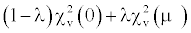(1)

Where 0 ≤ λ ≤ 1, χ2ν(0) denotes the central chi-square pdf on ν>0 degrees of freedom (df), and χ2ν(μ) denotes the chi-square pdf on ν df, with non-centrality parameter μ ≥ 0. We assume that ν is known, while λ and μ are unknown. We refer to (1) as the Contaminated Chi-square (CCS) model, since we regard χ2ν(0) as being contaminated by χ2ν(μ).

In this paper, we present a convenient procedure for testing

H0: λμ=0 versus H1: λμ>0,           (2)

We analyze its asymptotic and finite-sample properties, and we propose estimators of these parameters in the event that H0 is rejected. For a reason that will become apparent later, we refer to H0 as the omnibus null hypothesis. The CCS model simplifies to χ2ν(0), if and only if the omnibus null hypothesis is true.

To understand how the CCS model and omnibus null hypothesis relate to large-scale ANOVA testing, suppose that a microarray experiment [4,5] is performed to measure expression levels on each of n genes for subjects in independent samples of sizes g1, g2, …, gk from K populations. For gene i (1 ≤ i ≤ n), a one-way ANOVA may be conducted to test the genewise null hypothesis of equal mean expression levels across the K populations. This one-way ANOVA yields a test statistic Fi that has a central F distribution on (K-1) numerator and (g1+g2+… +gk-K) denominator df, under the genewise null hypothesis.

Let Xi denote the rescaled test statistic (K-1) Fi. With large (g1+g2+… +gk-K), Xi is distributed approximately χ2k-1(0) under the genewise null hypothesis, and approximately χ2k-1(μ), under the genewise alternative hypothesis, for some μ. We explain this approximation in the Appendix. If g1, g2, …, gk are not large enough to warrant this approximation, then a more sophisticated approach may be employed to transform F statistics into chi-square statistics; one such approach is described in and used for our case study in section 7.

Letting λ denote the proportion of genes for which mean expression levels are not equal across the K populations, we may regard the collection of rescaled test statistics X1, X2, …, Xn as a sample from the CCS model with ν=(K-1). If mean expression levels are equal across the K populations for all genes, then the CCS model reduces to χ2k-1(0). This is why λμ=0 is referred to as the omnibus null hypothesis.

The CCS model may also be applied and the omnibus null hypothesis tested, using subsets of X1, X2, …, Xn corresponding to biologically meaningful partitions of the genes. For example, suppose that n=2000 and that the first 1900 genes correspond to autosomes, while the last 100 genes correspond to sex chromosomes . Suppose, moreover, that there are g1=10 male subjects with a severe form of a disease suspected to be sex-linked, g2=10 male subjects with a mild form of the same disease, and g3=10 healthy male subjects. In this case, an investigator may wish to fit the CCS model separately to the first 1900 genes and to the last 100 genes.

If X1, X2, …, X1900 lead to rejection of the omnibus null hypothesis, then the investigator may question whether the disease is in fact sexlinked.

Otherwise, the investigator may justifiably discard the first 1900 genes and focus attention on the last 100. In particular, multiplicity adjustments for controlling Type I errors on genewise null hypotheses [7-9], can be based on the 100 remaining tests, instead of on the original 2000. Less stringent multiplicity adjustments will reduce Type II errors on the 100 remaining tests. Dai and Charnigo [10,11] have previously referred to this concept as gene filtration, although their earlier work did not consider the CCS model.

The CCS model may potentially be applied in other scenarios involving large numbers of tests. For instance, we envisage that the CCS model may be employed to analyze data on copy number variation , or transcript splicing variation . Before presenting our testing and estimation procedures (Section 5), we briefly review some literature on mixture modeling (Section 4). This review is not exhaustive but provides some context for this paper, allowing a more explicit articulation of this paper’s contributions. The remainder of this paper features empirical investigations, including both simulations (Section 6), and an application to real data (Section 7), as well as a discussion highlighting extensions of the ideas contained herein (Section 8). An appendix explains the rescaling of F statistics into approximate chi-square statistics.

#### Background on Mixture Modeling

Mixture modeling has been applied to interesting problems in disciplines, as varied as epidemiology [14,15], astronomy [16,17], biochemistry [18,19], and genetics [20,21].

From a technical perspective, mixture modeling is challenging because the usual regularity conditions for likelihood-based inference are not satisfied, when one is testing the number of components in a mixture model [22,23]. In particular, the asymptotic null distribution of a likelihood ratio test statistic for the number of components corresponds, under mild assumptions, to the supremum of a squared truncated Gaussian process defined on a compact parameter space [24-27].

Although likelihood-based inference is still possible via bootstrapping , or random field theory , more convenient approaches have been developed for many scenarios. These include Modified Likelihood Ratio (MLR) tests and estimators [30,31], Expectation Maximization (EM) tests and estimators [32,33], D tests [34,35] and moment-based tests .

Allison et al.  proposed applying a beta mixture model to the p-values from genewise hypothesis tests in a microarray experiment. This motivated Dai and Charnigo  to present MLR and D tests, for whether a beta mixture model for the p-values could be simplified to a uniform distribution. Subsequently, Dai and Charnigo  proposed applying a normal mixture model to the Z scores from genewise hypothesis tests (perhaps obtained by transforming T statistics), and developed tests for whether the normal mixture model could be simplified to a normal distribution. Whether looking at p-values or Z scores, an investigator could incorporate genewise hypothesis tests into a filtration algorithm.

The present work differs from the preceding efforts in that chi-square statistics (perhaps obtained by transforming F statistics) are now the focus, instead of p-values or Z scores. There are two reasons for this focus. First, while some microarray data analyses compare two populations on mean expression levels, other microarray data analyses compare more than two populations. An example, considered in our case study, appears in Blalock et al. , who compared three populations based on age strata to identify genes related to aging and cognition. Since ANOVA does not yield a Z score, the methodology of Dai and Charnigo  is inapplicable to such a scenario. However, the methodology proposed herein is applicable. In fact, the methodology proposed herein is still applicable when only two populations are compared, since a Z score may be converted to a chi-square statistic via squaring.

Second, a beta mixture model for p-values may differ from a uniform distribution in a way that is not indicative of systematic differential expression. For instance, 0.5 Beta(1,1)+0.5 Beta(2,0.5) corresponds to an excess of large p-values, rather than of small p-values. The tests of Dai and Charnigo  will detect an excess in either direction. Thus, the power to detect a specific alternative that is indicative of systematic differential expression may be lower than desired. The test proposed herein overcomes that limitation by rejecting the omnibus null hypothesis in (2), only when there is an excess of large chi-square statistics (or, equivalently, small p-values). Indeed, (2) makes explicit that the alternative to the omnibus null hypothesis is one-sided. As such, the test proposed herein may have better power to detect systematical differential expression than the tests of Dai and Charnigo .

#### Testing and Estimation Procedures

Suppose that X1, X2, …, Xn are a random sample from the CCS model (1). Our procedure for testing the omnibus null hypothesis in (2) is an intersection-union test based on the method of moments. More specifically, let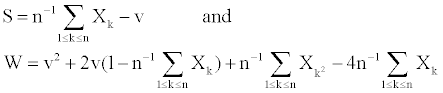(3)

Then S converges in probability to λμ, and W converges in probability to λμ2, by the Weak Law of Large Numbers and Slutsky’s Theorem. (If one wished to estimate λμp for a generic positive integer p, then one could derive an estimator using the first p moments; or if both S>0 and W>0, then one might estimate λμp by Wp-1S2-p. However, neither theorem 1 nor theorem 2 below involves estimation of λμp, so we do not discuss such estimation further).

The preceding considerations motivate us to reject the omnibus null hypothesis if S>scrit and W>wcrit, where scrit and wcrit are chosen to achieve the desired type I error probability. Theorem 1 below indicates how scrit and wcrit may be chosen. Before stating theorem 1, we establish some notation.

Let Φ denote the standard normal cumulative distribution function, and zc, the c quantile of the same. Let rj denote the jth moment of χ2ν(0) for 1 ≤ j ≤ 4, R the 2×2 matrix, whose ijth entry is ri+j-ri rj, and B the 2×2 matrix, whose first column is (1,0), and whose second column is (-2ν-4,1).

Theorem 1: Let 0<δ ≤ 1 and 0<ε ≤ 1 satisfy δε =α. Under the omnibus null hypothesis,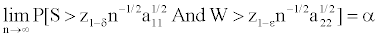(4)

Where a11 and a22 are the diagonal entries of the 2×2 matrix A=BTRB.

Moreover, under any fixed alternative (λ,μ)=(c1,c2), with 0<c1 ≤ 1 and c2>0,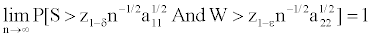(5)

Proof: Under the omnibus null hypothesis,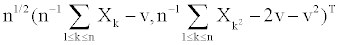converges in law to the multivariate normal distribution, with mean vector (0,0)T and covariance matrix R by the Central Limit Theorem. Then, (S,W)T converges in law to the multivariate normal distribution, with mean vector (0,0)T and covariance matrix A by Cramer’s Theorem. The key observation is that the off-diagonal entries of A are 0, hence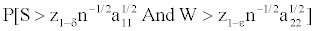converges to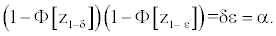Under the fixed alternative (λ,μ)=(c1,c2), S converges in probability to c1c2>0, and W converges in probability to
c1c2 2>0, so that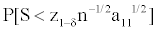and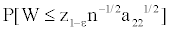converge to 0. Since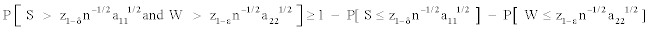,
the former must converge to 1. QED.

A few comments are in order. First, one may choose ε=1 (i.e. choose wcrit=-∞), and effectively base the test on only S, rather than on both S and W. In this case, one may replace z1-δ n-1/2 a111/2 by n-1 qνn,1-α-ν, where qνn,1-α denotes the 1-α quantile of χ2νn(0). Then the type I error probability is exactly α, for all finite n, not just converging to α in the limit. However, a potential problem with this choice is that one may reject the omnibus null hypothesis, when W<0. Since W is a moment-based estimator of λμ2, moment-based estimation of λ and μ, when W<0 leads to the estimator of λ, and/or that of μ, not belonging to the appropriate parameter space. However, a remedy is indicated in the next comment.

Second, choosing ε < ½ and δ < ½ (i.e., choosing wcrit>0 and scrit>0) guarantees that λ and μ may be estimated using moments, when the omnibus null hypothesis is rejected. This is described in theorem 2 and its corollary below. More specific choices of ε and δ can be recommended based on power considerations. However, while S and W are asymptotically independent under the omnibus null hypothesis, they may be correlated when the omnibus null hypothesis is false. Thus, analytically evaluating the power, in relation to ε and δ is difficult. However, we can gain some insights from simulation studies, which we pursue in section 6.

Third, in contrast with a likelihood ratio test for the number of components in a mixture model, the testing procedure of theorem 1 does not require a compact parameter space; note that no upper bound for μ was assumed. Moreover, the critical value is known, and thus, need not be estimated via resampling or random field theory. On the other hand, the problem in (2) is not, strictly speaking, determining the number of components in a mixture model. This is because, although (1) reduces to one component under the omnibus null hypothesis, (1) also reduces to one component, when λ=1 and μ>0.

Now, we address the estimation of λ and μ. Theorem 2 shows that, when the omnibus null hypothesis is false, S2/W and W/S are n1/2- consistent estimators of λ and μ, respectively. To state theorem 2, we introduce some more notations. Let mj=E[ X1j ] for 1 ≤ j ≤ 4, M the 2×2 matrix, whose ijth entry is mi+j-mi mj, and D the 2×2 matrix whose first column is

((m1-ν)(2m2-4m1-2νm1), -(m1 - ν)2)T/(m2+2ν+ν2- 4m1-2νm1)2 and whose second column is (-m2+2ν+ν2, m1-ν)T/ (m1-ν)2.

Theorem 2: Under any fixed alternative (λ,μ)=(c1,c2), with 0<c1≤ 1 and c2>0,

n1/2(S2/W-c1,W/S-c2)T converges in law to the multivariate normal distribution, with mean vector (0,0)T and covariance matrix DT M D.

Proof: By the Central Limit Theorem,converges in law to the multivariate normal distribution, with mean vector (0,0)T and covariance matrix M. The desired result then follows from Cramer’s Theorem. QED.

Although the probability that S<0 or W<0 is nonzero (in which case the estimator of λ, and/or that of μ will not belong to the appropriate parameter space), with ε ≤ ½ and δ ≤ ½, this event is a subset of accepting the omnibus null hypothesis. Hence, if one agrees to take ε ≤ ½ and δ ≤ ½, as well as to estimate λ and μ, only if the omnibus null hypothesis is rejected, then this event will not be encountered in practice. The following corollary, an immediate consequence of (5) from theorem 1, also demonstrates that such an agreement does not disturb the conclusion of theorem 2.

Corollary: Under any fixed alternative (λ,μ)=(c1,c2) with 0<c1 ≤ 1 and c2>0, the conditional distribution of n1/2 (S2/W-c1,W/S-c2)T, given that W>wcrit and S>scrit converges to the multivariate normal distribution, with mean vector (0, 0)T and covariance matrix DT M D.

#### Simulation Studies

To assess the type I and type II error rates of our testing procedure in finite samples, we conducted a number of simulation studies. In figure 1 and in the following text, we use this shorthand:

Figure 1: Displayed are rejection rates for omnibus null hypotheses in the simulation studies. Methods CCS1, CCS2, and CCS3, refer to our proposed testing procedure with (δ,ε)=(1/2, 1/10), (δ, ε)=(0.051/2,0.051/2), and (δ,ε)=(1/10, 1/2), respectively. Method CB refers to a modified likelihood ratio test applied to p-values, and treated as arising from the contaminated beta model .

* “CCS 1”: The procedure for testing the omnibus null hypothesis in (2) is applied directly to a random sample X1, X2, …, Xn from the CCS model (1), with δ=1/2 and ε=1/10. These choices of δ and ε emphasize W over S for rejection of the omnibus null hypothesis, requiring only that the latter be positive.

* “CCS 2”: Proceed as above, but with δ=ε=0.051/2. These choices emphasize W and S equally.

* “CCS 3”: Proceed as above, but with δ=1/10 and ε=1/2. These choices of δ and ε emphasize S over W for rejection of the omnibus null hypothesis, requiring only that the latter be positive.

* “CB”: A random sample X1, X2, …, Xn from the CCS model (1) is transformed by the survival function of the central chi-square distribution on ν df to yield “p-values” P1, P2 …, Pn. These are treated as if they had arisen from the Contaminated Beta (CB) model with pdf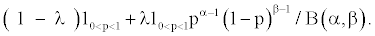(6)

The MLR test is applied to P1, P2 …, Pn to see whether the CB model can be reduced to a uniform distribution .

For each n in {50, 100, 250, 500, 1000}, we generated 10,000 random samples X1, X2, …, Xn from the CCS model (1) with λμ=0. Each random sample X1, X2, …, Xn was meant to mimic a collection of chi-square statistics, corresponding to n genes with no differential expression. We calculated type I error rates as the numbers of omnibus null hypothesis rejections divided by 10,000. The calculated type I error rates are displayed in the top left panel of figure 1. For methods CCS1, CCS2, and CCS3, these are between 0.0504 and 0.0613 at all n. Thus, the critical values for our testing procedure, which were based on the asymptotic result of theorem 1, appear satisfactory for finite samples. For method CB, the calculated type I error rates decrease from 0.0701 at n=50 to 0.0338 at n=1000, indicating that the MLR test applied to p-values is slightly anticonservative for small n.

We then generated 10,000 random samples, with λ=0.2 and μ=1. Each random sample was meant to mimic a collection of chi-square statistics, corresponding to a mix of differentially expressed genes (20%), with non differentially expressed genes (80%). Power, calculated as the number of omnibus null hypothesis rejections divided by 10,000, is displayed in the top right panel of figure 1. As anticipated, power increases with n for each method. Method CCS3 exhibits better power than method CCS2, which in turn is more powerful than method CCS1. Method CB appears relatively strong for large n, but comparatively weak for small n.

The remaining panels of figure 1 present power for (λ,μ)=(0.4,1), (λ,μ)=(0.2,2), (λ,μ)=(0.4,2), and (λ,μ)=(0.2,3), respectively. All of these scenarios maintain the relative ordering of methods CCS3, CCS2, and CCS1. Roughly speaking, method CB fares well with larger λ, μ, and n, but does not perform as well with smaller λ, μ, and n.

Based on the results of these simulation studies, we recommend taking δ=1/10 and ε=1/2, when applying our testing procedure. If n is large, or if λ and μ are anticipated to be large, then one may also wish to consider transforming chi-square statistics to p-values and then analyzing p-values using the CB model (6). However, the case study in section 7 will provide an important caveat, namely that a naïve analysis of p-values may lead to an inappropriate declaration of systematic differential expression. Thus, care must be exercised in any decision to transform chi-square statistics to p-values.

We also note that, while convenient to use because no resampling is required to ascertain critical values, our moment-based procedure for testing the omnibus null hypothesis in (2) may be less powerful than other approaches yet to be developed. In particular, we plan to investigate in a future manuscript whether the EM test [32,33], can be adapted to this setting. If so, then transforming chi-square statistics to p-values, and then analyzing p-values using the CB model (6) may become even less appealing.

#### Case Study

Dai and Charnigo  applied the CB model (6) to analyze the p-values generated from a microarray experiment conducted by Blalock et al. . Briefly, gene expression levels were acquired from the hippocampal tissue of 30 male Fischer rats divided into three groups of 10: “old”, “middle-aged”, and “young”. For each of 8799 genes, a one-way ANOVA was conducted to compare expression levels across the three groups. This produced 8799 F statistics, which in turn yielded the p-values. As noted by Dai and Charnigo , Blalock et al.  employed a three-step process to filter the p-values. In each step, genes were either retained for or eliminated from further consideration.

A major concern emerged when Dai and Charnigo  analyzed the p-values and, in particular, employed the MLR test , and D test , to see whether the CB model could be reduced to a uniform distribution. For the genes eliminated at step 3, the MLR test and D test decisively rejected the omnibus null hypothesis of a uniform distribution. However, the fitted model had λ=0.696, α=1.01, and β=1.28. Since α>1 does not correspond to an excess of small p-values, the departure from a uniform distribution may not indicate differential expression, but rather, as suggested by Allison et al. , correlations among the p-values corresponding to different genes. Thus, the alternative to the omnibus null hypothesis of a uniform distribution may be too broad if our main interest is in ascertaining differential expression.

With this concern in mind, we revisited these data. However, instead of analyzing p-values, we examined chi-square statistics. Since the denominator df for the underlying F statistics was not particularly large, we modified the F statistics based on the probability integral transformation , a more sophisticated approach than the rescaling described in section 1, and also consistent with the manner in which Dai and Charnigo  transformed T statistics to Z scores. More specifically, we converted the F statistics to chi-square statistics by successively applying the cumulative distribution function (cdf) of the central F distribution on 2 and 27 df, followed by the inverse cdf of the central chi-square distribution on 2 df.

Figure 2 shows histograms of chi-square statistics for all 8799 genes, for the genes eliminated in steps 1 and 2, and for the genes remaining after each step. Superimposed against each histogram are the fitted CCS model from (1), for which parameter estimates are displayed in table 1, and the null model χ22(0). In all six panels of figure 2, though most noticeably in the last panel, the fitted model yields a smaller density between 0 and 2, but a larger density between 5 and 10 compared to the null model. Overall, each fitted model is in much better concordance with its respective histogram than the null model, although even the fitted model overstates the number of very small chi-square statistics.

Figure 2: Shown are histograms of chi-square statistics for all 8799 genes in the case study for the genes eliminated in steps 1 and 2 of the filtration process employed by Blalock et al. , and for the genes remaining after each of the three steps. Superimposed against each histogram are the fitted CCS model for which parameter estimates are displayed in table 1, and the null model χ22(0).

 Genes Estimated λ Estimated μ all 8799 0.231 3.25 remaining after step 1 0.236 4.13 eliminated in step 1 0.389 1.28 remaining after step 2 0.223 4.54 eliminated in step 2 0.314 2.77 remaining after step 3 0.308 5.19

Table 1: Parameter Estimates for the CCS Model.

Correspondingly, our procedure for testing the omnibus null hypothesis in (2) yields a p-value less than 0.0001 for the omnibus null hypothesis, regardless of whether one defines this p-value by taking δ=1/2, ε=2α (i.e. p-value is half the smallest ε, at which the omnibus null hypothesis is rejected when δ is fixed at 1/2), or δ=ε=α1/2 (i.e. p-value is the square of the smallest ε, at which the omnibus null hypothesis is rejected when δ and ε are constrained to equality) or δ=2α, ε=α1/2(i.e. p-value is half the smallest δ, at which the omnibus null hypothesis is rejected when ε is fixed at 1/2).

The top panel of figure 3 shows a histogram of chi-square statistics for the 1483 genes eliminated in step 3, along with the null model χ22(0). No fitted CCS model is shown because in the notation of section 5, we have W<0. This precludes valid moment-based estimation of λ and μ. Although a likelihood-based approach to estimating λ and μ could be employed, this is not called for because the omnibus null hypothesis is not rejected at any α ≤ 0.25, regardless of whether one takes δ=1/2, ε=2α or δ=ε=α1/2, or δ=2α, ε=1/2. In fact, the null model is not a bad fit to the histogram, except for overstating the number of very small chi-square statistics. (Recall that the fitted CCS models in figure 2 had the same difficulty.)

Figure 3: The top panel shows a histogram of chi-square statistics for the 1483 genes eliminated in step 3 of the filtration process employed by Blalock et al. , along with the null model χ22(0). No fitted CCS model is shown, as the omnibus null hypothesis is not rejected at any α ≤ 0.25. The bottom panel shows a histogram of the p-values for these same 1483 genes, along with the fitted CB model, and the null model of a uniform distribution.

The bottom panel of figure 3 shows a histogram of the p-values for these same 1483 genes, along with the fitted CB model (6), and the null model of a uniform distribution. The fitted CB model is not suggestive of differential expression, as there is no marked surplus of small p-values. However, there are noticeably fewer extremely large p-values than would be compatible with a uniform distribution, and for this reason, both the MLR test and D test decisively reject the omnibus null hypothesis of a uniform distribution. This rejection is inappropriate in so far as one uses it to infer differential expression.

In summary, employing the CCS model to analyze chi-square statistics, instead of the CB model to assess p-values resolves the aforementioned concern, because the omnibus null hypothesis from (2) is not rejected for the genes eliminated in step 3. Thus, using the CCS model avoided an inappropriate declaration of differential expression.

#### Discussion

We have developed a convenient procedure for testing the omnibus null hypothesis of no contamination of a central chi-square distribution by a non-central chi-square distribution. This procedure is based on the first two sample moments, which permits critical values to be derived from quantiles of the standard normal distribution. Our simulation studies show that, even for small sample sizes, there is excellent agreement between the nominal and actual significance levels. In sharp contrast with likelihood ratio tests for mixture models, the asymptotic null distribution is uncomplicated [24-27], and thus there is no need for re-sampling , or random field theory , to obtain critical values.

As a follow-up to rejection of the omnibus null hypothesis, we have also proposed moment-based estimators of the contamination fraction and non-centrality parameter of the contaminating distribution. Provided that the quantities in question are both nonzero, our estimators are n1/2-consistent. Moreover, with suitable choices of δ and ε in the testing procedure, our estimators have probability 1 of being positive, conditional on rejection of the omnibus null hypothesis. This result is remarkable because moment-based estimators in mixture models ordinarily do not belong to their respective parameter spaces with probability 1, as noted by Charnigo et al.  for another type of contamination model.

Our testing and estimation procedures are primarily motivated by the modeling of numerous chi-square statistics arising from microarray data analysis specifically or large-scale testing generally. Such modeling expedites a filtration process, which, if successful, can reduce type II errors by justifying lighter controls for type I errors. While this filtration process was advocated by Dai and Charnigo  for the analysis of p-values, our case study provides a clear caveat against naïve analyses of p-values, and illustrates a real-world scenario in which analyzing chi-square statistics avoids an inappropriate declaration of differential expression. Moreover, our simulation studies show that under certain conditions, analysis of chi-square statistics may actually yield better power to detect differential expression than analysis of p-values.

While we have envisaged applying the CCS model to chi-square statistics monotonically related to F statistics from one-way ANOVA, the potential applications of the CCS model are considerably broader. For example, if the normality and equal variance assumptions underlying one-way ANOVA are untenable, then one may employ the nonparametric Kruskal-Wallis test for equal medians. Since the Kruskal-Wallis test statistic is distributed approximately χ2K-1(0) when the medians are equal, the CCS model can be applied in conjunction with chi-square statistics from Kruskal-Wallis tests, as easily as with F statistics from one-way ANOVA.

Moreover, sophisticated experimental designs or sampling schemes may preclude using either one-way ANOVA or Kruskal-Wallis tests. For instance, Mao et al.  obtained multiple tissue samples from some of their subjects, so that linear mixed models were required to test genewise null hypotheses. However, as long as genewise null hypotheses are tested using chi-square or F statistics (or even Z or T statistics, since these can be squared), the CCS model remains applicable.

A number of promising avenues exist for future research. One of them is to investigate whether the EM test [32,33], can be profitably employed in the setting of the CCS model, and if so, whether power to reject a false omnibus null hypothesis is improved. Our simulation studies suggest that there may indeed be room for improvement, as the procedure proposed herein was not uniformly more powerful than the MLR test applied to p-values derived from the chi-square statistics.

Another topic for future research is to generalize the CCS model to provide greater flexibility for describing real data. For instance, suppose that each Xi has its own non-centrality parameter μi under the genewise alternative hypothesis. Then we may consider a new model,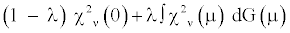(7)

Where ∫ denotes integration and G is some cumulative distribution function defined on the nonnegative real numbers. Note that the first sample moment of data from (7) is ν, if and only if (3) reduces to χ2ν(0), as both are equivalent to λ{1-G(0)}=0. Thus, one obtains a consistent level α test for whether (7) reduces to χ2ν(0), by asking whether the first sample moment exceeds n-1 qνn,1-α. However, the subsequent estimation of λ and G are anticipated to be considerably more delicate.

#### Appendix

We now explain our statement from section 1 that a rescaled F statistic may be regarded as an approximate chi-square statistic. Suppose that Y1 has the central chi-square distribution on ν1 df, and that independently, Y2 has the central chi-square distribution on ν2 df. Then, the quotient (Y11)/(Y22) has the central F distribution on ν1 numerator and ν2 denominator df .

Since Y2 has mean ν2 and variance 2ν2, Chebychev’s Inequality implies that (Y22) converges in probability to 1 as ν2→∞. Thus, when ν2 is large, (Y22)≈1, and so (Y11)/(Y22) ≈ (Y11). In other words, a quantity with a central F distribution on large denominator df resembles a chi-square random variable divided by the numerator df.

To make explicit the connection to our statement from section 1, let Y1 be the between sum of squares from a one-way ANOVA divided by the underlying variance of the individual observations, let ν1=K-1 be the corresponding df, let Y2 be the within sum of squares divided by the underlying variance, and let v2=g1+g2+…+gk-K be the corresponding df. Put F=(Y11)/( Y22). If g1+g2+…+gk-K is sufficiently large, then (K-1)F ≈ Y1.

From a practical perspective, one may decide whether g1+g2+…+gk-K is sufficiently large by evaluating whether P[|log(Y22)| ≥ tol ] ≤ tol, where tol is a specified tolerance. One may interpret tol as the maximum acceptable Levy distance between the cumulative distribution functions of log[(K-1)F] and log[Y1]. As such, we recommend setting tol no larger than 0.20, and preferably as small as 0.10. Corresponding to these choices, one has g1+g2+…+gk-K ≥ 83 andg1+g2+…+gk-K ≥ 543, respectively. Since g1+g2+…+gk-K=27 in the case study of section 7, we did not use rescaling in that case study, but instead relied on a more sophisticated approach for transforming F statistics into chi-square statistics.

#### References

Select your language of interest to view the total content in your interested language

### Article Usage

• Total views: 12547
• [From(publication date):
January-2013 - Dec 06, 2019]
• Breakdown by view type
• HTML page views : 8712Can't read the image? click here to refresh